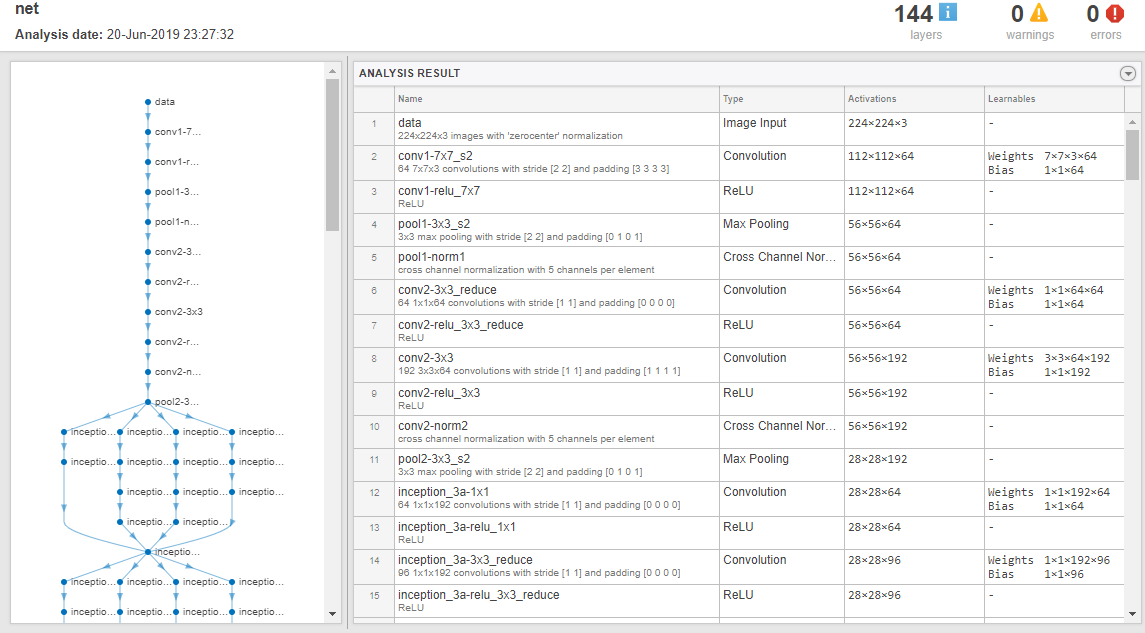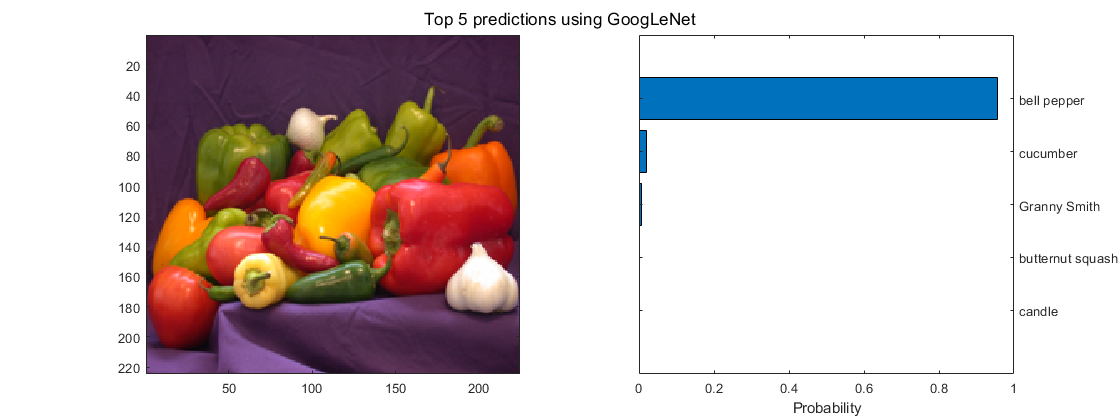Code Generation for Deep Learning Networks by Using cuDNN

With GPU Coder™, you can generate optimized code for prediction of a variety of trained deep learning networks from Deep Learning Toolbox™. The generated code implements the deep convolutional neural network (CNN) by using the architecture, the layers, and parameters that you specify in the input SeriesNetwork (Deep Learning Toolbox) or DAGNetwork (Deep Learning Toolbox) object. The code generator takes advantage of NVIDIA® CUDA® deep neural network library (cuDNN) for NVIDIA GPUs. cuDNN is a GPU-accelerated library of primitives for deep neural networks. The generated code can be integrated into your project as source code, static or dynamic libraries, or executables that you can deploy to a variety of NVIDIA GPU platforms.

Generate code for convolutional networks by using one of the methods:

• The standard codegen function that generates CUDA code from a MATLAB® entry-point function.

• The GPU Coder app that generates CUDA code from a MATLAB entry-point function.

Note

In previous releases you could target the cuDNN library by using the cnncodegen function. From R2021a onwards, the cnncodegen function generates C++ code and make files for only the ARM® Mali GPU processor.

Generate Code and Classify Images by Using GoogLeNet

In this example, you use GPU Coder to generate CUDA code for the pretrained googlenet (Deep Learning Toolbox) deep convolutional neural network and classify an image. GoogLeNet has been trained on over a million images and can classify images into 1000 object categories (such as keyboard, coffee mug, pencil, and animals). The network has learned rich feature representations for a wide range of images. The network takes an image as input, and then outputs a label for the object in the image together with the probabilities for each of the object categories. This example show you how to generate code for the pretrained network by using the codegen command and the GPU Coder app.

Requirements

Required

This example generates CUDA MEX that has the following additional requirements.

1. Deep Learning Toolbox.

2. Deep Learning Toolbox Model for GoogLeNet Network support package.

3. GPU Coder Interface for Deep Learning Libraries support package.

4. CUDA enabled NVIDIA GPU and a compatible driver. For 8-bit integer precision, the CUDA GPU must have a compute capability of 6.1, 6.3 or higher.

Optional

For non-MEX builds such as static, dynamic libraries, or executables, this example has the following additional requirements.

1. CUDA toolkit and cuDNN libraries. For information on the supported versions of the compilers and libraries, see Installing Prerequisite Products.

2. Environment variables for the compilers and libraries. For more information, see Environment Variables.

1. Load the pretrained GoogLeNet network. You can choose to load a different pretrained network for image classification. If you do not have the required support packages installed, the software provides a download link.

2. The object net contains the DAGNetwork object. Use the analyzeNetwork (Deep Learning Toolbox) function to display an interactive visualization of the network architecture, to detect errors and issues in the network, and to display detailed information about the network layers. The layer information includes the sizes of layer activations and learnable parameters, the total number of learnable parameters, and the sizes of state parameters of recurrent layers.

analyzeNetwork(net);3. The image that you want to classify must have the same size as the input size of the network. For GoogLeNet, the size of the imageInputLayer (Deep Learning Toolbox) is 224-by-224-by-3. The Classes property of the output classificationLayer (Deep Learning Toolbox) contains the names of the classes learned by the network. View 10 random class names out of the total of 1000.

classNames = net.Layers(end).Classes;
numClasses = numel(classNames);
disp(classNames(randperm(numClasses,10)))

'speedboat'
'window screen'
'isopod'
'wooden spoon'
'lipstick'
'drake'
'hyena'
'dumbbell'
'strawberry'
'custard apple'

For more information, see List of Deep Learning Layers (Deep Learning Toolbox).

Create an Entry-Point Function

1. Write an entry-point function in MATLAB that:

1. Uses the coder.loadDeepLearningNetwork function to load a deep learning model and to construct and set up a CNN class. For more information, see Load Pretrained Networks for Code Generation.

2. Calls predict (Deep Learning Toolbox) to predict the responses.

2. For example:

persistent mynet;

if isempty(mynet)
end

% pass in input
out = predict(mynet,in);

A persistent object mynet loads the DAGNetwork object. At the first call to the entry-point function, the persistent object is constructed and set up. On subsequent calls to the function, the same object is reused to call predict on inputs, avoiding reconstructing and reloading the network object.

Note

Code generation requires the network to be loaded into a persistent object.

3. You can also use the activations (Deep Learning Toolbox) method to network activations for a specific layer. For example, the following line of code returns the network activations for the layer specified in layerIdx.

out = activations(mynet,in,layerIdx,'OutputAs','Channels');

4. You can also use the classify (Deep Learning Toolbox) method to predict class labels for the image data in in using the trained network, mynet.

[out,scores] = classify(mynet,in);

For LSTM networks, you can also use the predictAndUpdateState (Deep Learning Toolbox) and resetState (Deep Learning Toolbox) methods. For usage notes and limitations of these method, see the corresponding entry in the Supported Functions table.

Code Generation by Using codegen

1. To configure build settings such as output file name, location, and type, you create coder configuration objects. To create the objects, use the coder.gpuConfig function. For example, when generating CUDA MEX using the codegen command, use cfg = coder.gpuConfig('mex');

Other available options are:

1. cfg = coder.gpuConfig('lib');, to create a code generation configuration object for use with codegen when generating a CUDA C/C++ static library.

2. cfg = coder.gpuConfig('dll');, to create a code generation configuration object for use with codegen when generating a CUDA C/C++ dynamic library.

3. cfg = coder.gpuConfig('exe');, to create a code generation configuration object for use with codegen when generating a CUDA C/C++ executable.

2. To specify code generation parameters for cuDNN, set the DeepLearningConfig property to a coder.CuDNNConfig object that you create by using coder.DeepLearningConfig.

cfg = coder.gpuConfig('mex');
cfg.TargetLang = 'C++';
cfg.DeepLearningConfig = coder.DeepLearningConfig('cudnn');
cfg.DeepLearningConfig.AutoTuning = true;
cfg.DeepLearningConfig.DataType = 'fp32';

Specify the precision of the inference computations in supported layers by using the DataType property. When performing inference in 32-bit floats, use 'fp32'. For 8-bit integer, use 'int8'. Default value is 'fp32'. INT8 precision requires a CUDA GPU with minimum compute capability of 6.1. Use the ComputeCapability property of the GpuConfig object to set the appropriate compute capability value.

Note

Code generation for INT8 data type does not support multiple deep learning networks in the entry-point function.

When performing inference in INT8 precision using cuDNN version 8.1.0, issues in the NVIDIA library may cause significant degradation in performance.

3. Run the codegen command. The codegen command generates CUDA code from the googlenet_predict.m MATLAB entry-point function.

codegen -config cfg googlenet_predict -args {ones(224,224,3)} -report

1. The -report option instructs codegen to generate a code generation report that you can use to debug your MATLAB code.

2. The -args option instructs codegen to compile the file googlenet_predict.m by using the class, size, and complexity specified for the input in. The value (224,224,3) corresponds to input layer size of the GoogLeNet network.

3. The -config option instructs codegen to use the specified configuration object for code generation.

Note

You can specify half-precision inputs for code generation. However, the code generator type casts the inputs to single-precision. The Deep Learning Toolbox uses single-precision, floating-point arithmetic for all computations in MATLAB.

The code generator uses column-major layout by default. To use row-major layout pass the -rowmajor option to the codegen command. Alternatively, configure your code for row-major layout by modifying the cfg.RowMajor parameter in the code generation configuration object.

4. When code generation is successful, you can view the resulting code generation report by clicking View Report in the MATLAB Command Window. The report is displayed in the Report Viewer window. If the code generator detects errors or warnings during code generation, the report describes the issues and provides links to the problematic MATLAB code. See Code Generation Reports.

Code generation successful: View report

Generated Code

The DAG network is generated as a C++ class containing an array of 78 layer classes. The code generator reduces the number of layers by using layer fusion optimization of convolutional and ReLU layers. A snippet of the class declaration from googlenet_predict_types.h file is shown.

• The setup() method of the class sets up handles and allocates memory for each layer of the network object.

• The predict() method invokes prediction for each of the 78 layers in the network.

• The DeepLearningNetwork.cu file contains the definitions of the object functions for the b_googlenet_0 class.

Binary files are exported for layers with parameters such as fully connected and convolution layers in the network. For instance, files cnn_googlenet_conv*_w and cnn_googlenet_conv*_b correspond to weights and bias parameters for the FusedConvReLU layers in the network. The code generator places these binary files in the codegen folder.

Note

On Windows® systems, some antivirus software such as Bit Defender can incorrectly identify some weight files as infected and delete them. These cases are false positives and the files can be marked as safe in your antivirus program.

In the generated code file googlenet_predict.cu, the entry-point function googlenet_predict() constructs a static object of b_googlenet_0 class type and invokes setup and predict on this network object.

Generate Code by Using the App

To specify the entry-point function and specifying input types, complete the procedure in the app. See Code Generation by Using the GPU Coder App.

In the Generate Code step:

1. Set the Build type to MEX.

2. Click . In the Deep Learning pane, set Target library to cuDNN.3. Close the settings window. To generate CUDA code, click .

Generated Makefile

For 'lib', 'dll', and 'exe' targets, the code generator creates the *_rtw.mk make file in the codegen folder. In this make file, the location of the generated code is specified by using the START_DIR variable found in the MACROS section. By default, this variable points to the path of the current working folder where the code is generated. If you plan to move the generated files and use the makefile to build, replace the generated value of START_DIR with the appropriate path location.

Run the Generated MEX

1. The image that you want to classify must have the same size as the input size of the network. Read the image that you want to classify and resize it to the input size of the network. This resizing slightly changes the aspect ratio of the image.

inputLayerSize = net.Layers(1).InputSize;
im = imresize(im,inputLayerSize(1:2));

2. Call GoogLeNet predict on the input image.

3. Display the top five predicted labels and their associated probabilities as a histogram. Because the network classifies images into so many object categories, and many categories are similar, it is common to consider the top-five accuracy when evaluating networks. The network classifies the image as a bell pepper with a high probability.

[scores,indx] = sort(predict_scores, 'descend');
classNamesTop = classNames(indx(1:5));

h = figure;
h.Position(3) = 2*h.Position(3);
ax1 = subplot(1,2,1);
ax2 = subplot(1,2,2);

image(ax1,im);
barh(ax2,scores(5:-1:1))
xlabel(ax2,'Probability')
yticklabels(ax2,classNamesTop(5:-1:1))
ax2.YAxisLocation = 'right';